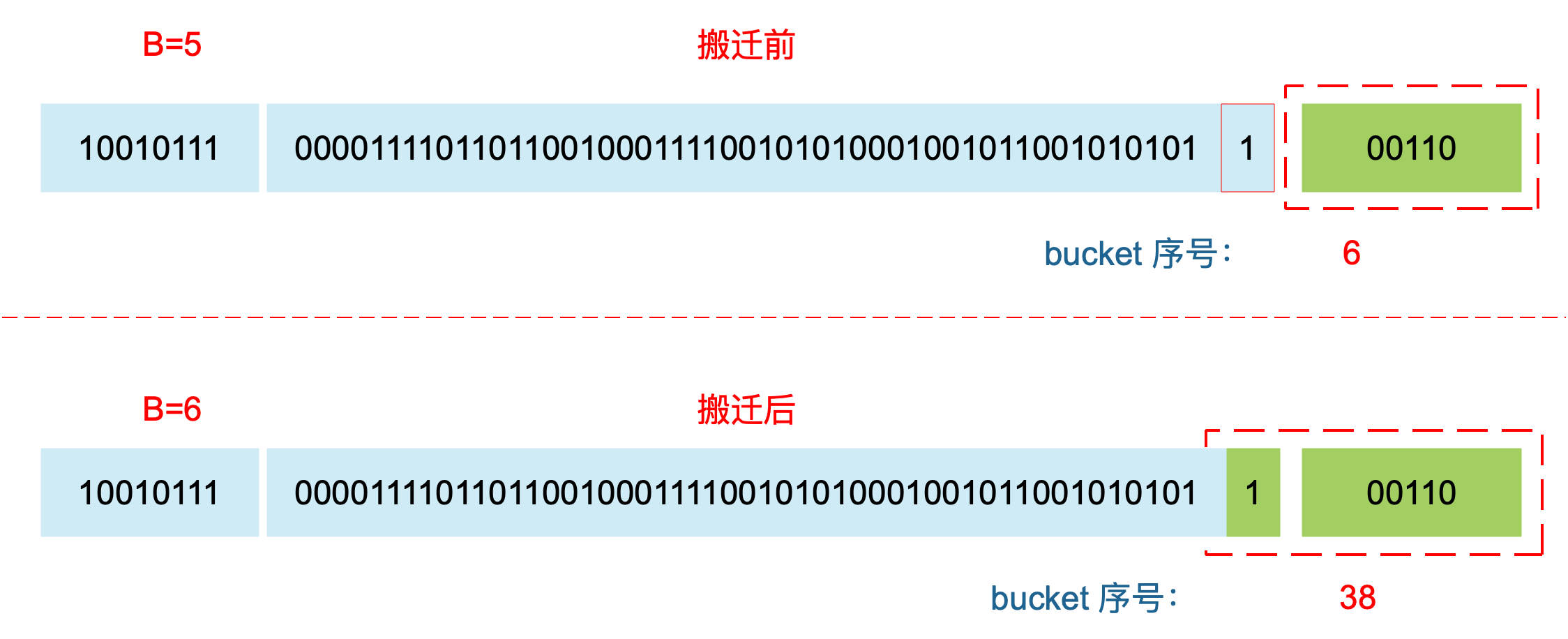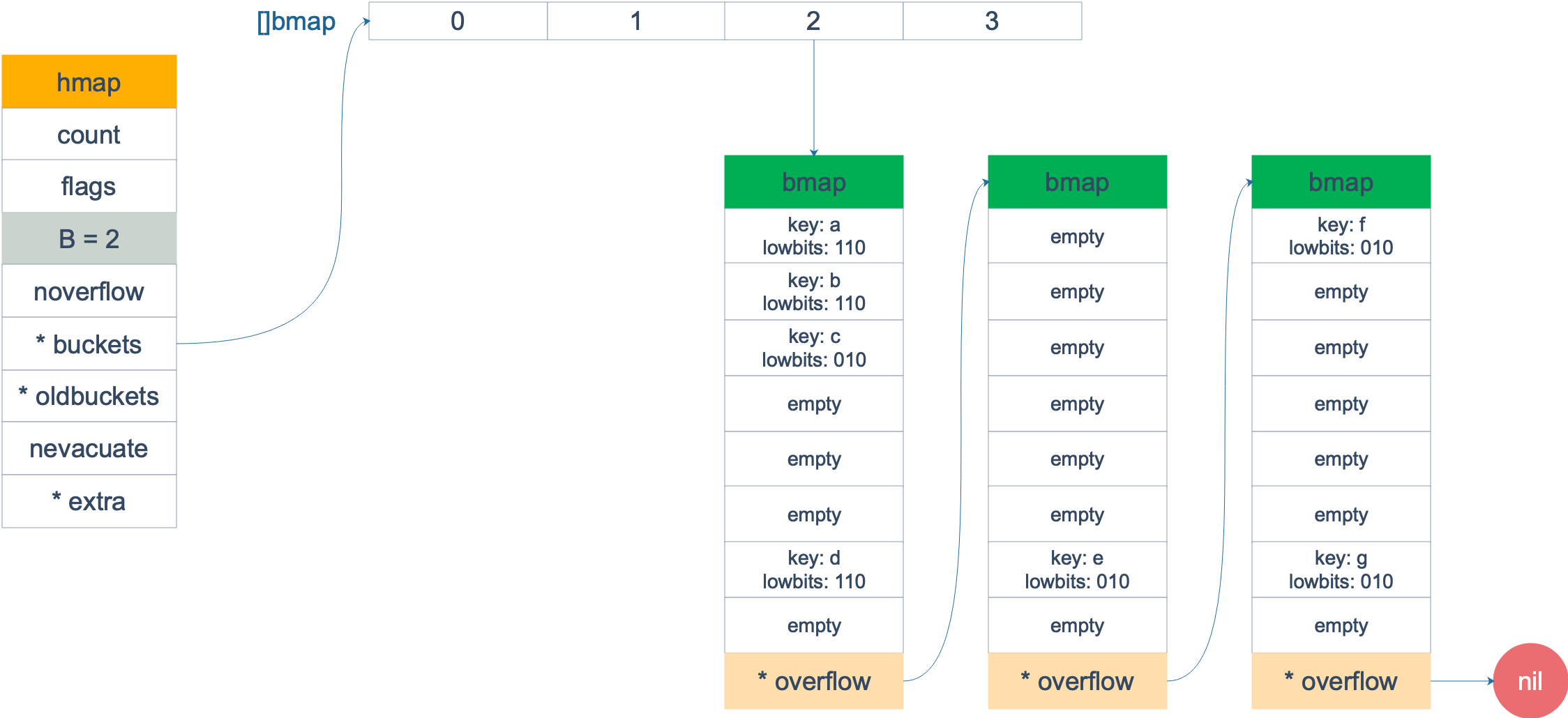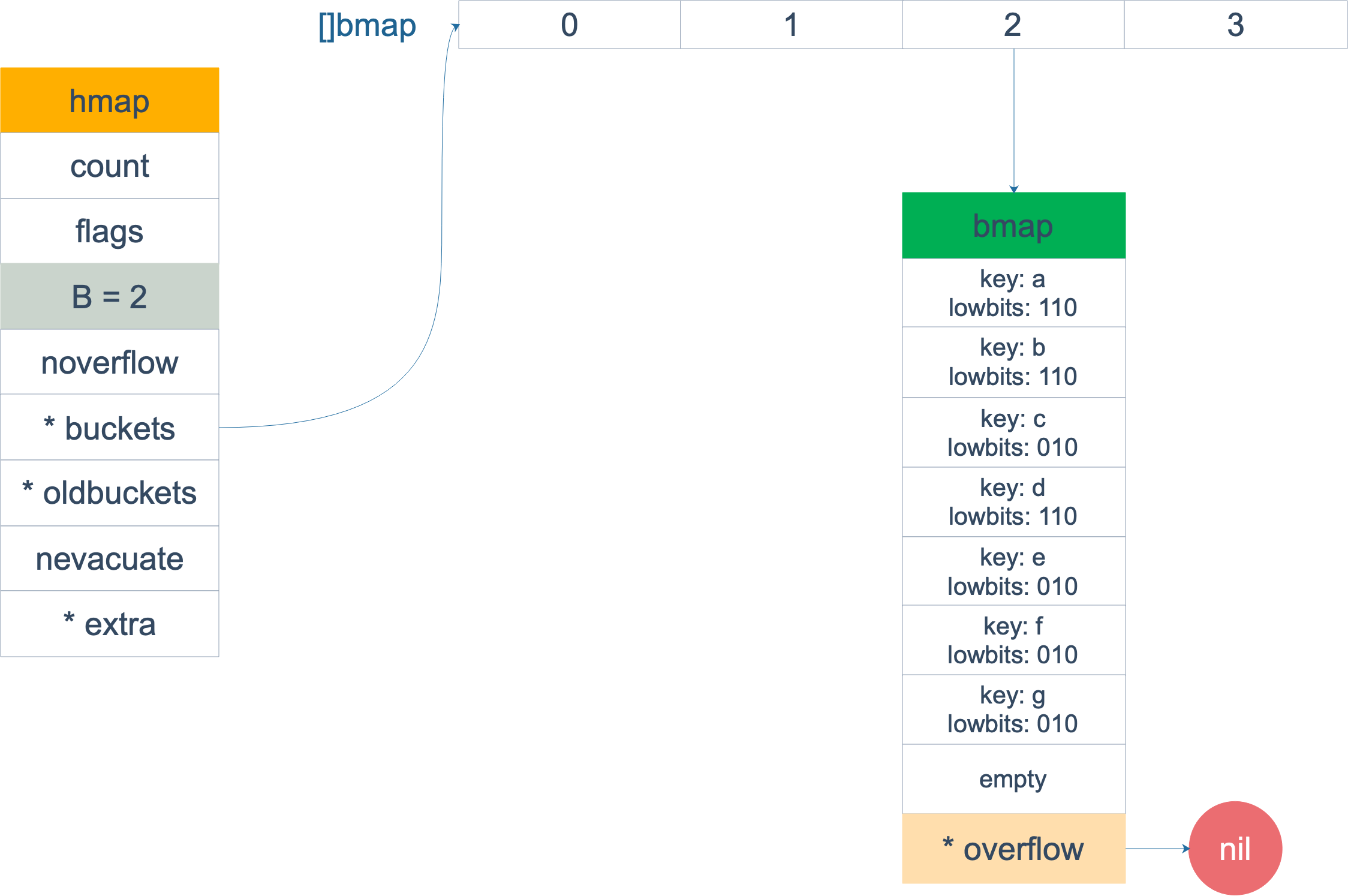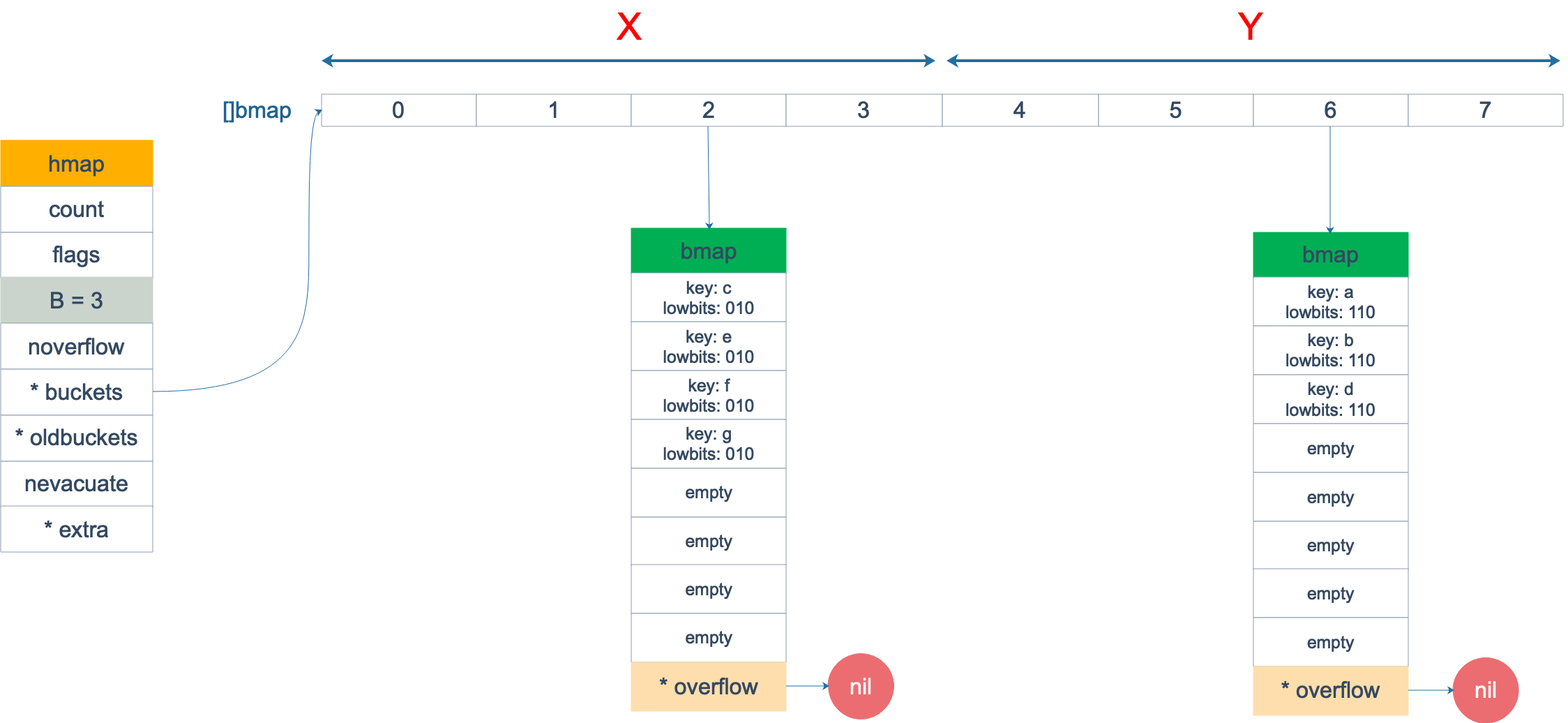Go 语言采用一个 bucket 里装载 8 个 key，定位到某个 bucket 后，还需要再定位到具体的 key，这实际上又用了时间换空间。

 1  loadFactor := count / (2^B) 

count 就是 map 的元素个数，2^B 表示 bucket 数量。

1. 装载因子超过阈值，源码里定义的阈值是 6.5。
2. overflow 的 bucket 数量过多：当 B 小于 15，也就是 bucket 总数 2^B 小于 2^15 时，如果 overflow 的 bucket 数量超过 2^B；当 B >= 15，也就是 bucket 总数 2^B 大于等于 2^15，如果 overflow 的 bucket 数量超过 2^15。

  1 2 3 4 5 6 7 8 9 10 11 12 13 14 15 16 17 18 19  // src/runtime/hashmap.go/mapassign // 触发扩容时机 if !h.growing() && (overLoadFactor(int64(h.count), h.B) || tooManyOverflowBuckets(h.noverflow, h.B)) { hashGrow(t, h) } // 装载因子超过 6.5 func overLoadFactor(count int64, B uint8) bool { return count >= bucketCnt && float32(count) >= loadFactor*float32((uint64(1)<= uint16(1)<= 1<<15 } 

  1 2 3 4 5 6 7 8 9 10 11 12 13 14 15 16 17 18 19 20 21 22 23 24 25 26 27 28 29 30 31  func hashGrow(t *maptype, h *hmap) { // B+1 相当于是原来 2 倍的空间 bigger := uint8(1) // 对应条件 2 if !overLoadFactor(int64(h.count), h.B) { // 进行等量的内存扩容，所以 B 不变 bigger = 0 h.flags |= sameSizeGrow } // 将老 buckets 挂到 buckets 上 oldbuckets := h.buckets // 申请新的 buckets 空间 newbuckets, nextOverflow := makeBucketArray(t, h.B+bigger) flags := h.flags &^ (iterator | oldIterator) if h.flags&iterator != 0 { flags |= oldIterator } // 提交 grow 的动作 h.B += bigger h.flags = flags h.oldbuckets = oldbuckets h.buckets = newbuckets // 搬迁进度为 0 h.nevacuate = 0 // overflow buckets 数为 0 h.noverflow = 0 // …… } 

 1 2 3 4  flags := h.flags &^ (iterator | oldIterator) if h.flags&iterator != 0 { flags |= oldIterator } 

 1 2 3  x = 01010011 y = 01010100 z = x &^ y = 00000011 

 1 2 3 4 5 6 7 8  // 可能有迭代器使用 buckets iterator = 1 // 可能有迭代器使用 oldbuckets oldIterator = 2 // 有协程正在向 map 中写入 key hashWriting = 4 // 等量扩容（对应条件 2） sameSizeGrow = 8 

 1 2 3 4 5 6 7 8 9  func growWork(t *maptype, h *hmap, bucket uintptr) { // 确认搬迁老的 bucket 对应正在使用的 bucket evacuate(t, h, bucket&h.oldbucketmask()) // 再搬迁一个 bucket，以加快搬迁进程 if h.growing() { evacuate(t, h, h.nevacuate) } } 

h.growing() 函数非常简单：

 1 2 3  func (h *hmap) growing() bool { return h.oldbuckets != nil } 

bucket&h.oldbucketmask() 这行代码，如源码注释里说的，是为了确认搬迁的 bucket 是我们正在使用的 bucket。oldbucketmask() 函数返回扩容前的 map 的 bucketmask。

  1 2 3 4 5 6 7 8 9 10 11 12 13 14 15 16 17 18 19 20 21 22 23 24 25 26 27 28 29 30 31 32 33 34 35 36 37 38 39 40 41 42 43 44 45 46 47 48 49 50 51 52 53 54 55 56 57 58 59 60 61 62 63 64 65 66 67 68 69 70 71 72 73 74 75 76 77 78 79 80 81 82 83 84 85 86 87 88 89 90 91 92 93 94 95 96 97 98 99 100 101 102 103 104 105 106 107 108 109 110 111 112 113 114 115 116 117 118 119 120 121 122 123 124 125 126 127 128 129 130 131 132 133 134 135 136 137 138 139 140 141 142 143 144 145 146 147 148 149 150 151 152 153 154 155 156 157 158 159 160 161 162 163 164 165 166 167 168 169 170 171 172 173 174 175 176 177 178 179 180 181 182 183 184 185 186 187  func evacuate(t *maptype, h *hmap, oldbucket uintptr) { // 定位老的 bucket 地址 b := (*bmap)(add(h.oldbuckets, oldbucket*uintptr(t.bucketsize))) // 结果是 2^B，如 B = 5，结果为32 newbit := h.noldbuckets() // key 的哈希函数 alg := t.key.alg // 如果 b 没有被搬迁过 if !evacuated(b) { var ( // 表示bucket 移动的目标地址 x, y *bmap // 指向 x,y 中的 key/val xi, yi int // 指向 x，y 中的 key xk, yk unsafe.Pointer // 指向 x，y 中的 value xv, yv unsafe.Pointer ) // 默认是等 size 扩容，前后 bucket 序号不变 // 使用 x 来进行搬迁 x = (*bmap)(add(h.buckets, oldbucket*uintptr(t.bucketsize))) xi = 0 xk = add(unsafe.Pointer(x), dataOffset) xv = add(xk, bucketCnt*uintptr(t.keysize))、 // 如果不是等 size 扩容，前后 bucket 序号有变 // 使用 y 来进行搬迁 if !h.sameSizeGrow() { // y 代表的 bucket 序号增加了 2^B y = (*bmap)(add(h.buckets, (oldbucket+newbit)*uintptr(t.bucketsize))) yi = 0 yk = add(unsafe.Pointer(y), dataOffset) yv = add(yk, bucketCnt*uintptr(t.keysize)) } // 遍历所有的 bucket，包括 overflow buckets // b 是老的 bucket 地址 for ; b != nil; b = b.overflow(t) { k := add(unsafe.Pointer(b), dataOffset) v := add(k, bucketCnt*uintptr(t.keysize)) // 遍历 bucket 中的所有 cell for i := 0; i < bucketCnt; i, k, v = i+1, add(k, uintptr(t.keysize)), add(v, uintptr(t.valuesize)) { // 当前 cell 的 top hash 值 top := b.tophash[i] // 如果 cell 为空，即没有 key if top == empty { // 那就标志它被"搬迁"过 b.tophash[i] = evacuatedEmpty // 继续下个 cell continue } // 正常不会出现这种情况 // 未被搬迁的 cell 只可能是 empty 或是 // 正常的 top hash（大于 minTopHash） if top < minTopHash { throw("bad map state") } k2 := k // 如果 key 是指针，则解引用 if t.indirectkey { k2 = *((*unsafe.Pointer)(k2)) } // 默认使用 X，等量扩容 useX := true // 如果不是等量扩容 if !h.sameSizeGrow() { // 计算 hash 值，和 key 第一次写入时一样 hash := alg.hash(k2, uintptr(h.hash0)) // 如果有协程正在遍历 map if h.flags&iterator != 0 { // 如果出现 相同的 key 值，算出来的 hash 值不同 if !t.reflexivekey && !alg.equal(k2, k2) { // 只有在 float 变量的 NaN() 情况下会出现 if top&1 != 0 { // 第 B 位置 1 hash |= newbit } else { // 第 B 位置 0 hash &^= newbit } // 取高 8 位作为 top hash 值 top = uint8(hash >> (sys.PtrSize*8 - 8)) if top < minTopHash { top += minTopHash } } } // 取决于新哈希值的 oldB+1 位是 0 还是 1 // 详细看后面的文章 useX = hash&newbit == 0 } // 如果 key 搬到 X 部分 if useX { // 标志老的 cell 的 top hash 值，表示搬移到 X 部分 b.tophash[i] = evacuatedX // 如果 xi 等于 8，说明要溢出了 if xi == bucketCnt { // 新建一个 bucket newx := h.newoverflow(t, x) x = newx // xi 从 0 开始计数 xi = 0 // xk 表示 key 要移动到的位置 xk = add(unsafe.Pointer(x), dataOffset) // xv 表示 value 要移动到的位置 xv = add(xk, bucketCnt*uintptr(t.keysize)) } // 设置 top hash 值 x.tophash[xi] = top // key 是指针 if t.indirectkey { // 将原 key（是指针）复制到新位置 *(*unsafe.Pointer)(xk) = k2 // copy pointer } else { // 将原 key（是值）复制到新位置 typedmemmove(t.key, xk, k) // copy value } // value 是指针，操作同 key if t.indirectvalue { *(*unsafe.Pointer)(xv) = *(*unsafe.Pointer)(v) } else { typedmemmove(t.elem, xv, v) } // 定位到下一个 cell xi++ xk = add(xk, uintptr(t.keysize)) xv = add(xv, uintptr(t.valuesize)) } else { // key 搬到 Y 部分，操作同 X 部分 // …… // 省略了这部分，操作和 X 部分相同 } } } // 如果没有协程在使用老的 buckets，就把老 buckets 清除掉，帮助gc if h.flags&oldIterator == 0 { b = (*bmap)(add(h.oldbuckets, oldbucket*uintptr(t.bucketsize))) // 只清除bucket 的 key,value 部分，保留 top hash 部分，指示搬迁状态 if t.bucket.kind&kindNoPointers == 0 { memclrHasPointers(add(unsafe.Pointer(b), dataOffset), uintptr(t.bucketsize)-dataOffset) } else { memclrNoHeapPointers(add(unsafe.Pointer(b), dataOffset), uintptr(t.bucketsize)-dataOffset) } } } // 更新搬迁进度 // 如果此次搬迁的 bucket 等于当前进度 if oldbucket == h.nevacuate { // 进度加 1 h.nevacuate = oldbucket + 1 // Experiments suggest that 1024 is overkill by at least an order of magnitude. // Put it in there as a safeguard anyway, to ensure O(1) behavior. // 尝试往后看 1024 个 bucket stop := h.nevacuate + 1024 if stop > newbit { stop = newbit } // 寻找没有搬迁的 bucket for h.nevacuate != stop && bucketEvacuated(t, h, h.nevacuate) { h.nevacuate++ } // 现在 h.nevacuate 之前的 bucket 都被搬迁完毕 // 所有的 buckets 搬迁完毕 if h.nevacuate == newbit { // 清除老的 buckets h.oldbuckets = nil // 清除老的 overflow bucket // 回忆一下： 表示当前 overflow bucket //  表示 old overflow bucket if h.extra != nil { h.extra.overflow = nil } // 清除正在扩容的标志位 h.flags &^= sameSizeGrow } } } 

evacuate 函数的代码注释非常清晰，对着代码和注释是很容易看懂整个的搬迁过程的，耐心点。evacuate 函数每次只完成一个 bucket 的搬迁工作，因此要遍历完此 bucket 的所有的 cell，将有值的 cell copy 到新的地方。bucket 还会链接 overflow bucket，它们同样需要搬迁。因此会有 2 层循环，外层遍历 bucket 和 overflow bucket，内层遍历 bucket 的所有 cell。这样的循环在 map 的源码里到处都是，要理解透了。

  1 2 3 4 5 6 7 8 9 10 11 12  if top&1 != 0 { // top hash 最低位为 1 // 新算出来的 hash 值的 B 位置 1 hash |= newbit } else { // 新算出来的 hash 值的 B 位置 0 hash &^= newbit } // hash 值的 B 位为 0，则搬迁到 x part // 当 B = 5时，newbit = 32，二进制低 6 位为 10 0000 useX = hash&newbit == 0